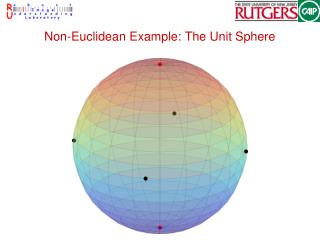Download PresentationNon-Euclidean Example: The Unit Sphere

# Non-Euclidean Example: The Unit Sphere - PowerPoint PPT PresentationDownload Presentation## Non-Euclidean Example: The Unit Sphere

- - - - - - - - - - - - - - - - - - - - - - - - - - - E N D - - - - - - - - - - - - - - - - - - - - - - - - - - -
##### Presentation Transcript

1. Non-Euclidean Example: The Unit Sphere

2. Differential Geometry • Formal mathematical theory • Work with small ‘patches’ • the ‘patches’ look Euclidean • Do calculus over the patches

3. Manifolds • Open Sets • Coordinate neighbourhood • Compatible neighbourhoods

4. Tangents • Tangent Vectors • Tangent Space, • Inner Product • Norm: • depends on • varies smoothly

5. Geodesics and Metrics • The shortest path between two points is the geodesic • The length of the geodesic is the distance between the points

6. Exponential and Logarithm Maps • : Maps tangents to the manifold • : Maps points on the manifold to • Both maps are locally well defined

7. Gradient • In Euclidean space: direction of fastest increase • On a manifold: tangent of fastest increase • Definition: is a real valued function. The gradient at is satisfying directional derivative along delta

8. The Conversion Table • X. Pennec, P. Fillard and N. Ayache , “A Riemannian Framework for Tensor Computing,” International. Journal of Computer Vision., 66(1), 41–66, 2006.

9. Matrix Lie Groups • Sets of matrices which • form a group under matrix multiplication • are Riemannian manifolds • Examples • Rigid body transformations SE(n) • Rotations SO(n) • Affine motions A(n) • W. Rossman, “Lie Groups: An Introduction through Linear Groups,” Oxford University Press, 2003.

10. Grassmann Manifolds, . • Each point on the Grassmann manifold, , represents a dimensional subspace of . • Numerically, represented by an orthonormal basis • matrix such that • Representation is not unique • computation should account for this • A. Edelman, T. A. Arias and S. T. Smith, “The Geometry of Algorithms with Orthogonality Constraints,” SIAM Journal on Matrix Analysis and Applications, 20(2), 303–353, 1998.

11. The Essential Manifold • Set of matrices with • two equal and one zero singular value • let the two equal singular values be 1 • Equivalent to SO(3)xSO(3) • two-time covering of the essential manifold • Can also be expressed as a homogeneous space • S. Soatto, R. Frezza and P. Perona , “Recursive Estimation on the Essential Manifold,” 3rd Europan Conference on Computer Vision, Stockholm, Sweden, May 1994, vol.II, p.61-72.

12. Twisted Pairs

13. The Symmetric Manifold • contains symmetric positive definite matrices. e.g. diffusion tensor MRI • it has two different metrics • The Affine Invariant metric • The Log-Euclidean Metric • practically similar to the affine invariant metric • computationally easier to work with • V. Arsigny , P. Fillard, X. Pennec and N. Ayache , “Geometric Means in a Novel Vector Space Structure on Symmetric Positive-Definite Matrices,” SIAM Journal of Matrix Analysis and Applications, 29(1), 328–347, 2006.

14. Mean Shift for Euclidean Spaces • The kernel density estimate • Mean shift as normalized gradient of where • The iteration

15. Mean Shift • Gradient ascent on kernel density • but, no line search • Equivalent, to expectation-maximization • Nonparametric Clustering • D. Comaniciu and P. Meer , “Mean Shift: A Robust Approach Towards Feature Space Analysis,” IEEE Transactions on Pattern Analysis and Machine Intelligence, vol.24, 603–619, 2002. • D. Comaniciu, V. Ramesh and P. Meer , “Kernel-based Object Tracking,” IEEE Transactions on Pattern Analysis and Machine Intelligence, vol.25, 564–577, 2003.

16. Mean Shift for Manifolds • The kernel density estimate • Mean shift as normalized gradient of • The iteration

17. Mean Shift for Riemannian Manifolds • Map points to tangent space • Get weighted average of tangent vectors • this is the mean shift vector • Map the mean shift vector back to the manifold

18. Theoretical Properties • Gradient ascent on kernel density • Nonlinear mean shift is provably convergent • upper limit on allowed bandwidth • nonlinear mean shift is equivalent to EM • for homogeneous spaces

19. Motion Segmentation • Hypothesis Generation Lie groups • Randomly pick elemental subset • Generate parameter hypothesis • Clustering • Cluster parameters on the manifold • Return • Number of dominant modes • Positions of dominant modes

20. Affine Motion: Results A(2)

21. Camera Pose Estimation SE(3)

22. Camera Pose Estimation: Results

23. Factorization: Results G10,3

24. Essential Matrix: Results SO(3)xSO(3)

25. Discontinuity Preserving Filtering • An image is a mapping from a lattice in to data lying on a manifold • Filtering: Run mean shift in the space Iterations update spatial and parameter values. • If the iteration from converges to , set in the filtered image

26. Chromatic Noise Filtering M = G3,1

27. Chromatic Noise Filtering

28. DTI Images R3xSym+3